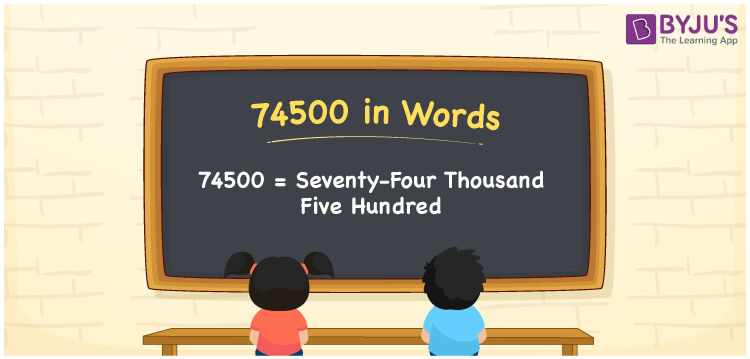# 74500 in Words

The word form of a number is nothing but the name of that number. For example, the name of the cardinal number 74500 is Seventy-four thousand five hundred, which is used to communicate the number to others. Therefore, we can write 74500 in words as Seventy-four thousand five hundred. Also, learn how to convert 74500 into words using a place value chart here in this article.

 74500 in words Seventy-four thousand five hundred Seventy-four thousand five hundred in Numbers 74500

## 74500 in English Words

We use the English alphabet to express numbers in words. So, we can spell 74500 in English words as “Seventy-four thousand five hundred”.## How to Write 74500 in Words?

The number 74500 has five digits. Thus, we need to create a place value chart with five columns to convert the number 74500 into words.

 Ten thousands Thousands Hundreds Tens Ones 7 4 5 0 0

Here, ones = 0, tens = 0, hundreds = 5, thousands = 4, ten thousands = 7

The above numbers can be expanded as:

7 × Ten thousand + 4 × Thousand + 5 × Hundred + 0 × Ten + 0 × One

= 7 × 10000 + 4 × 1000 + 5 × 100 + 0 × 10 + 0 × 1

= 70000 + 4000 + 500

= Seventy Thousand + Four thousand + Five hundred

= Seventy-four thousand five hundred

Therefore, 74500 in words = Seventy-four thousand five hundred.

74500 is a natural number that is the successor of 74499 and predecessor of 74501.

74500 in words – Seventy-four thousand five hundred

Is 74500 an odd number? – No

Is 74500 an even number? – Yes

Is 74500 a prime number? – No

Is 74500 a composite number? – Yes

Is 74500 a perfect square number? – No

Is 74500 a perfect cube number? – No

## Frequently Asked Questions on 74500 in Words

Q1

### How do you write 74500 in words?

We can write the number 74500 in words as Seventy-four thousand five hundred.
Q2

### Write Rs. 74500 in words.

The amount Rs. 74500 can be written in words as “Seventy-four thousand five hundred”.
Q3

### What is the number name for 74500?

The number name for 74500 is given as Seventy-four thousand five hundred.